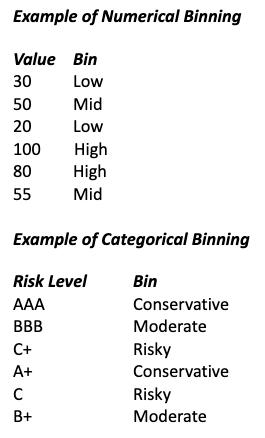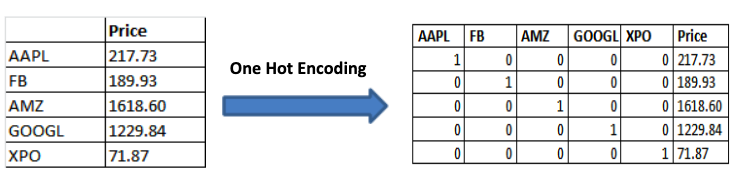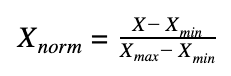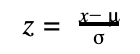# Data Preprocessing in Data Science and Machine Learning

Data preprocessing is where data scientist spent most of their time. These tasks involve selecting the appropriate features as well as clean and prepare them to become the inputs or independent variables in a machine learning model.

Model performance is strictly related with the selection and cleaning of the features.  Below we describe common tasks which are necessary to conduct before fitting and evaluate a model. These tasks will improve the accuracy of the model due to the increase of the inputs quality.

### Handling Data Types of the Features

In python data analysis, each column of a dataset is loaded into the environment with a specific data type. Most common data types are float, string, integer, datetime. In many occasion numerical data, is loaded as character or string type because could contains specific character that lead to interpret all column as a character column.

Likewise, the datetime fields if not have the correct format, is interpreted as strings or character object. Prior to start any analysis that uses dates is necessary to convert this variable into a datetime object.

To ensure the integrity of the data, one of the first steps is to explore the data types of a dataset in order to observe if they have the correct type.

### Handling Missing Data

Missing values are one of the common issues in machine learning models, and their causes are related to human errors, interruptions in the data flow, and incorrect measurements among others. Most of the algorithms in machine learning do not accept missing values and through errors if a dataset has missing values.

Therefore it is necessary to solve this issue utilizing some mechanism to deal or remove them. Removing rows or columns with missing data, can affect the performance of the model, as the size of the data decrease.

A more elegant method is the Numerical imputation of the median of the variable in places with missing values. Median is preferable to the mean because it is not affected by outliers. This solution would preserve the size of the data. In case of categorical values, missing values can be replaced with the most common categorical value of the column.

### Handling Outliers

For financial time series, outliers can be detected by plotting the distribution of the returns and observe if the distribution has extreme fat tails, which is a hint of some anomaly in the data.

Also outliers can be detected by using the percentiles of the data. Researchers can define certain percentage threshold in the upper-bottom of the distribution where values beyond these limits are considered outliers.

### Binning

Binning means group a numerical or categorical variable into bins.  In cases where categorical values have low frequency in a huge dataset, they can be binned into a category called “others”, which convert the model more robust.### Logarithmic Transformation

This technique is used in many statistical analysis and machine learning models, as the log remove the skewness of the data an approximate it distribution to a normal distribution. On the other hand, the log transformation of the variables decreases the effect of outliers.

### Encoding data

One of the most common encoding methods in machine learning is called One Hot Encoding. The method spread the values in a column to multiple columns, where the values in the original columns are used to rename the new columns .After the transformation the new columns takes two possible values that are 1 or 0. This method is mainly used with categorical variable and is similar to create dummy variables for each of the categorical values on a specific column.

### Scaling

After scaling a dataset, all the continuous variables become identical in terms of the range. This process is not critic for some algorithms, but there are algorithms such as K-means (Unsupervised technique)  that work with the distance measure, so it is required to scale all the inputs in order to have values that can be compared.

Some techniques to scale values on a dataset are Normalization and Standardization.

NormalizationNormalization scales all values in the range 0 and 1. Each value of the variable is subtracted by the min value and divided by the difference between the max value and the min value. The procedure does not change the distribution of the feature. Before normalization, outliers should be handled.

StandardizationThis method is also called the z-score and scale each of the values of a column by removing from them the mean of the column and divided by the standard deviation. This technique decreases the effect of outliers in each feature.